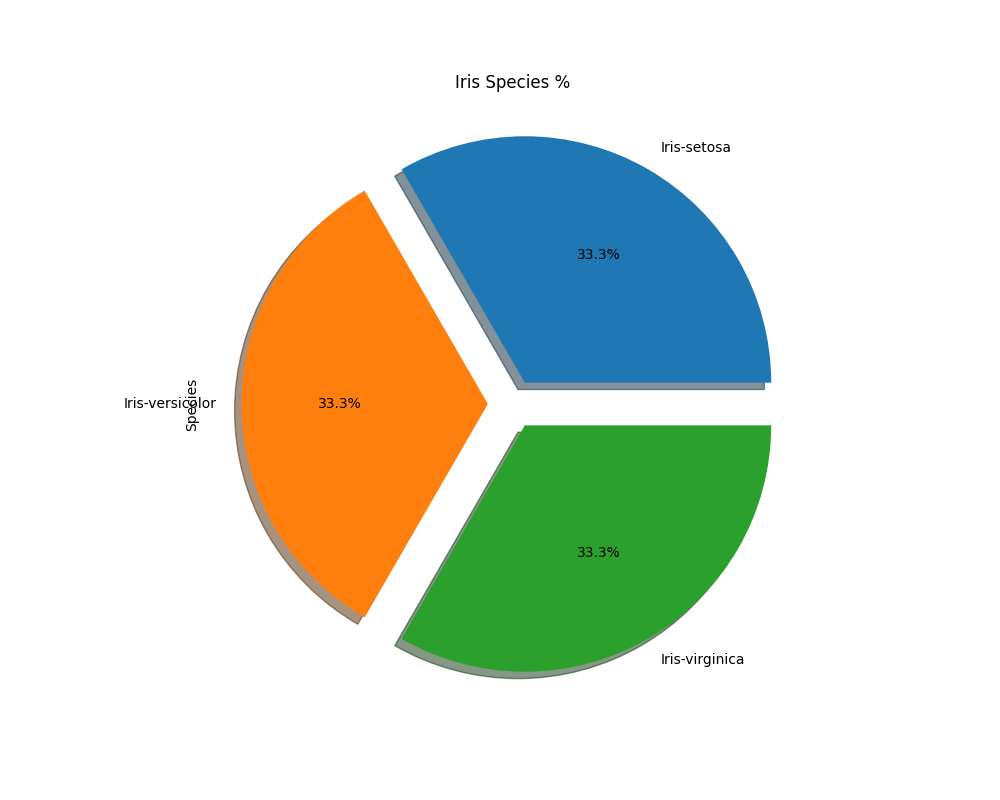﻿ Python Machine learning Scikit-learn: Create a Pie plot to get the frequency of the three species of the Iris data - w3resource# Python Scikit-learn: Create a Pie plot to get the frequency of the three species of the Iris data

## Python Machine learning Iris Visualization: Exercise-3 with Solution

Write a Python program to create a Pie plot to get the frequency of the three species of the Iris data.

Sample Solution:

Python Code:

``````import pandas as pd
import matplotlib.pyplot as plt
ax=plt.subplots(1,1,figsize=(10,8))
plt.title("Iris Species %")
plt.show()
```
```

Output:

``````

Python Code Editor:

Have another way to solve this solution? Contribute your code (and comments) through Disqus.

What is the difficulty level of this exercise?

﻿